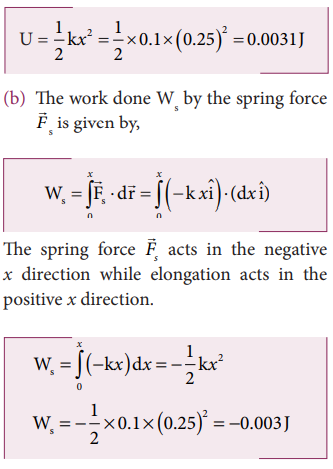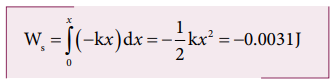Home | | Physics 11th std | Solved Example Problems for Elastic Potential Energy

# Solved Example Problems for Elastic Potential Energy

Physics : Work, Energy and Power : Elastic Potential Energy

Solved Example Problems for  Elastic Potential Energy

Example 4.9

Let the two springs A and B be such that kA>kB. On which spring will more work has to be done if they are stretched by the same force?

### SolutionThe work done on the springs are stored as potential energy in the springs.kA>kB implies that UB>UA . Thus, more work is done on B than A.

### Example 4.10

A body of mass m is attached to the spring which is elongated to 25 cm by an applied force from its equilibrium position.

a. Calculate the potential energy stored in the spring-mass system?

b. What is the work done by the spring force in this elongation?

c. Suppose the spring is compressed to the same 25 cm, calculate the potential energy stored and also the work done by the spring force during compression. (The spring constant, k = 0.1 N m-1).

### Solution

The spring constant, k = 0.1 N m-1

The displacement, x = 25 cm = 0.25 m

a. The potential energy stored in the spring is given byNote that the potential energy is defined through the work done by the external agency. The positive sign in the potential energy implies that the energy is transferred from the agency to the object. But the work done by the restoring force in this case is negative since restoring force is in the opposite direction to the displacement direction.

c. During compression also the potential energy stored in the object is the same.Work done by the restoring spring force during compression is given byIn the case of compression, the restoring spring force acts towards positive x-axis and displacement is along negative x direction.Study Material, Lecturing Notes, Assignment, Reference, Wiki description explanation, brief detail
11th Physics : UNIT 4 : Work, Energy and Power : Solved Example Problems for Elastic Potential Energy |